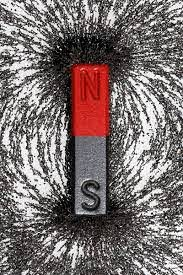## Iron, Magnet and Wall  Codechef November Long challenge 2020 solution with code explanation -

Iron, Magnet and Wall  Codechef November Long challenge 2020 solution with code explanation

Chef loves to play with iron (Fe) and magnets (Ma). He took a row of $N$ cells (numbered $1$ through $N$) and placed some objects in some of these cells. You are given a string $S$ with length $N$ describing them; for each valid $i$, the $i$-th character of $S$ is one of the following:

• 'I' if the $i$-th cell contains a piece of iron
• 'M' if the $i$-th cell contains a magnet
• '_' if the $i$-th cell is empty
• ':' if the $i$-th cell contains a conducting sheet
• 'X' if the $i$-th cell is blocked

If there is a magnet in a cell $i$ and iron in a cell $j$, the attraction power between these cells is ${P}_{i,j}=K+1-|j-i|-{S}_{i,j}$, where ${S}_{i,j}$ is the number of cells containing sheets between cells $i$ and $j$. This magnet can only attract this iron if ${P}_{i,j}>0$ and there are no blocked cells between the cells $i$ and $j$.

Chef wants to choose some magnets (possibly none) and to each of these magnets, assign a piece of iron which this magnet should attract. Each piece of iron may only be attracted by at most one magnet and only if the attraction power between them is positive and there are no blocked cells between them. Find the maximum number of magnets Chef can choose.

### Input

• The first line of the input contains a single integer $T$ denoting the number of test cases. The description of $T$ test cases follows.
• The first line of each test case contains two space-separated integers $N$ and $K$.
• The second line contains a single string $S$ with length $N$.

### Output

For each test case, print a single line containing one integer ― the maximum number of magnets that can attract iron.

### Constraints

• $1\le T\le 2,000$
• $1\le N\le {10}^{5}$
• $0\le K\le {10}^{5}$
• $S$ contains only characters 'I', 'M', '_', ':' and 'X'
• the sum of $N$ over all test cases does not exceed $5\cdot {10}^{6}$

Subtask #1 (30 points): there are no sheets, i.e. $S$ does not contain the character ':'

Subtask #2 (70 points): original constraints

### Example Input

2
4 5
I::M
9 10
MIM_XII:M


### Example Output

1
2


### Explanation

Example case 1: The attraction power between the only magnet and the only piece of iron is $5+1-3-2=1$. Note that it decreases with distance and the number of sheets.

Example case 2: The magnets in cells $1$ and $3$ can attract the piece of iron in cell $2$, since the attraction power is $10$ in both cases. They cannot attract iron in cells $6$ or $7$ because there is a wall between them.

The magnet in cell $9$ can attract the pieces of iron in cells $7$ and $6$; the attraction power is $8$ and $7$ respectively.### Solution-

To solve this problem you need to think some logic that .
1-none of iron and magnet can be paired if any wall is situated between them.
2- A iron/magnet can pair only with one magnet/iron.
3- to maximize the final answer you need need to pair a iron with a magnet which is farthest from iron. similarly you have to for magnet.

Actually implementation part is a little bit more tricky/ tough then logic.

Code explanation-
store index of iron, magnet, and wall.
and store count of the metal sheet between 0 to the current index.
and start pairing iron with the farthest magnet and magnet with the farthest iron.
If the wall encounters a clear index of iron magnet and wall.
And repeat the same process after the wall.

Note- This question can be done by another approach.
Follow this video for another approach.

Must Join Telegram channel for Editorial -